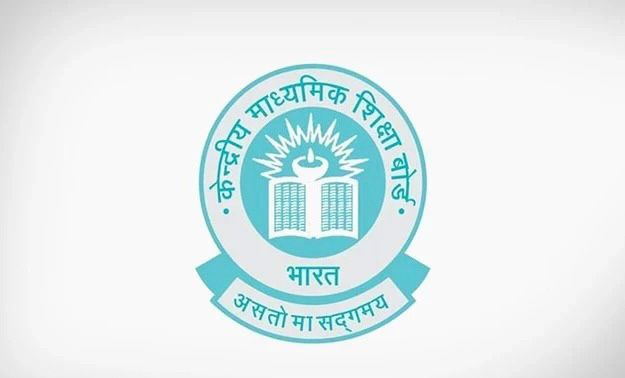# If A(3, √3), B(0, 0), and C(3, k) are the three vertices of an equilateral triangle ABC, then the value of k is

CBSE Maths Exam 2021-22 Term 1, question: If A(3, √3), B(0, 0), and C(3, k) are the three vertices of an equilateral triangle ABC, then the value of k isa) 2

b) -3

c) -√3

d) -√2